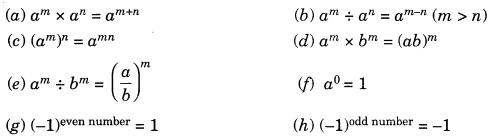On this page, you will find Exponents and Powers Class 7 Notes Maths Chapter 13 Pdf free download. CBSE NCERT Class 7 Maths Notes Chapter 13 Exponents and Powers will seemingly help them to revise the important concepts in less time.

## CBSE Class 7 Maths Chapter 9 Notes Exponents and Powers

### Exponents and Powers Class 7 Notes Conceptual Facts

1. Exponents are used to express the large numbers in shorter form to make them easier to read, compare the understand.

2. When a number is multiplied by itself several times, it can be expressed in short form as under

x x x x x x x x x = x5 which is called exponential expression.
x is called base and 5 is exponent or power or index.

3. In general an = a x a x a x a x … n times = an

4. Properties of exponents:

5. Any number raised to power 1 gives the same number.

6. For example: 51 = 5, 1001 = 100

7. A negative number raised to an odd positive integer is always negative.
For example: (-4)3 = (-4) x (-4) x (-4) = -64

8. A negative number raised to an even positive integer is always positive.
For example: (-3)4 = (-3) x (-3) x (-3) x (-3) = 81

9. A positive number raised to an even or odd integer is always positive.
For example:
24 = 2 x 2 x 2 x 2 = 16
= 33 x 3 x 3 = 27

10. Any number raised to power zero, it gives 1.
For example: (-5)° = 1, (1000)°= 1

11. Power 2 is also called square of.

12. Power 3 is also called cube of.

13. Laws of exponents: For any non-zero integers a and b and whole numbers m and